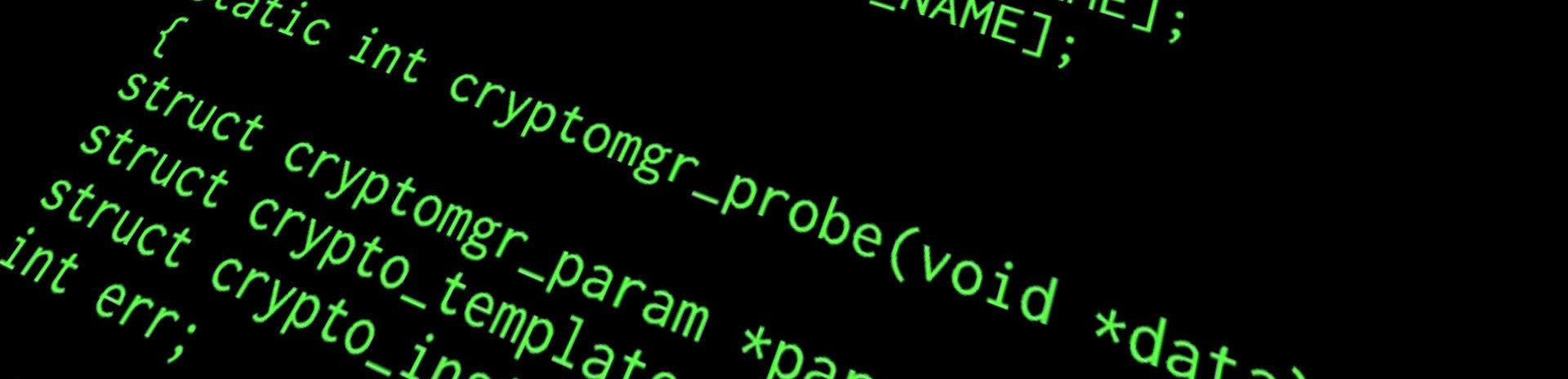Ben OliverHow to make a custom escaper for Twig & Symfony
"Make Twig output any format you like."
June 11 2017

If you use the Symfony Framwork with Twig for templating, and want to output files that are more than just html, css or javascript you’ve come to the right place! This guide uses LaTeX as an example but actually, you can swap out the characters for anything you like.

## What is a custom escaper?

Let’s say you have a twig template that pulls data from your database, it might look like this.

<html>
<body>
<p>Name: {{ array.name }}</p>
<p>Film Type: {{ array.film_type }}</p>
</body>
</html>


Twig fills in the bits in {{ }} with the data.

What if {{ array.film_type }} returned something like Black & White? It would break the html in some cases, because the & should really be &amp;. So if {{ array.name }} contained something like Ben is "cool" twig would convert it to Ben is &quot;cool&quot; so that the quotes don’t get dropped by the web browser and it displays properly. Google ‘escape characters’ for more info.

The escaper is basically the set of rules it uses to do this. So it says ‘find every " and change it to &quot;’. This is built in behaviour for html, but what if you need it to say something else? What if you wanted to convert & to and - or even not convert it at all? You need your own set of rules, or a ‘Custom Escaper’.

## Creating your escaper

### The service

Start by creating a service.


<?php

namespace AppBundle\FunBundle\Service;

class Latex
{

public function __construct($twig) {$this->twig = $twig; } public function escaper() { // Get twig env$twig = $this->twig; // Start setEscaper called 'latex' - call it what you want$twig->getExtension('Twig_Extension_Core')->setEscaper('latex',
function($twig,$string, $charset){ // Replace every instance of '&' with '\&'$string = str_replace("&", "\\&", $string); } return$string;
);
}
}



This is a very simple example I made for LaTeX, you can add you own lines to flesh it out. The full one I use is quite long, so I’ve posted it down at the bottom of the page.

Next you need to register that service with your app.


# app/config/services.yml
services:
app.latex:
class: AppBundle\FunBundle\Service\Latex
arguments: ['@twig']



With that done, all you need to do is call the service in your controller when you need it. So if you are about to render a Latex template in a controller, include the following:


$latexer =$this->get('app.latex');
$latexer->escaper();  Then you need to tell the template itself to look for LaTeX instead of html (the default). In your template file:  {% autoescape 'latex' %} # latex code goes here {% end autoescape %}  And you’re done! As I said, this can be done with anything really, you just need to set the rules in your service. The example above works for & only; below is the full code I actually use for LaTeX escaping. It includes <, >, #, ~ amongst others. I adapted it from this project.  <?php namespace AppBundle\FunBundle\Service; class Latex { // Get the global params public function __construct($twig)
{
$this->twig =$twig;
}

public function escaper()
{
$twig =$this->twig;
$twig->getExtension('Twig_Extension_Core')->setEscaper('latex', function($twig, $string,$charset){

// Try to replace HTML entities
preg_match_all('/&[a-zA-Z]+;/iu', $string,$matches);
foreach ($matches as$match) {
$string = str_replace($match, $this->htmlCodes[$match], $string); } // Special characters$string = str_replace('&sup2;', '\\textsuperscript{2}', $string);$string = str_replace('&sup3;', '\\textsuperscript{3}', $string);$string = str_replace('²', '\\textsuperscript{2}', $string);$string = str_replace('³', '\\textsuperscript{3}', $string); // Remove remaining HTML entities$string = preg_replace('/&[a-zA-Z]+;/iu', '', $string); // Adjust known characters$string = str_replace("ä", "\\\"a", $string);$string = str_replace("á", "\\'a", $string);$string = str_replace("à", "\\a", $string);$string = str_replace("Ä", "\\\"A", $string);$string = str_replace("Á", "\\'A", $string);$string = str_replace("À", "\\A", $string);$string = str_replace("ë", "\\\"e", $string);$string = str_replace("é", "\\'e", $string);$string = str_replace("è", "\\e", $string);$string = str_replace("ê", "\\^e", $string);$string = str_replace("Ë", "\\\"E", $string);$string = str_replace("É", "\\'E", $string);$string = str_replace("È", "\\E", $string);$string = str_replace("Ê", "\\^E", $string);$string = str_replace("ï", "\\\"i", $string);$string = str_replace("í", "\\'i", $string);$string = str_replace("ì", "\\i", $string);$string = str_replace("Ï", "\\\"I", $string);$string = str_replace("Í", "\\'I", $string);$string = str_replace("Ì", "\\I", $string);$string = str_replace("ö", "\\\"o", $string);$string = str_replace("ó", "\\'o", $string);$string = str_replace("ò", "\\o", $string);$string = str_replace("Ö", "\\\"O", $string);$string = str_replace("Ó", "\\'O", $string);$string = str_replace("Ò", "\\O", $string);$string = str_replace("õ", "\\~O", $string);$string = str_replace("Õ", "\\~O", $string);$string = str_replace("ü", "\\\"u", $string);$string = str_replace("ú", "\\'u", $string);$string = str_replace("ù", "\\u", $string);$string = str_replace("Ü", "\\\"U", $string);$string = str_replace("Ú", "\\'U", $string);$string = str_replace("Ù", "\\U", $string);$string = str_replace("ñ", "\\~n", $string);$string = str_replace("ß", "{\\ss}", $string);$string = str_replace("ç", "\\c{c}", $string);$string = str_replace("Ç", "\\c{C}", $string);$string = str_replace("ș", "\\c{s}", $string);$string = str_replace("Ș", "\\c{S}", $string);$string = str_replace("ŭ", "\\u{u}", $string);$string = str_replace("Ŭ", "\\u{U}", $string);$string = str_replace("ă", "\\u{a}", $string);$string = str_replace("Ă", "\\u{A}", $string);$string = str_replace("ă", "\\v{a}", $string);$string = str_replace("Ă", "\\v{A}", $string);$string = str_replace("š", "\\v{s}", $string);$string = str_replace("Š", "\\v{S}", $string);$string = str_replace("Ø", "{\\O}", $string);$string = str_replace("ø", "{\\o}", $string); // Remaining special characters (cannot be placed with the others, // as then the html entity replace would fail).$string = str_replace("#", "\\#", $string);$string = str_replace("_", "\\_", $string);$string = str_replace("^", "\\^{}", $string);$string = str_replace("°", "\$^{\\circ}\$", $string);$string = str_replace(">", "\\textgreater ", $string);$string = str_replace("<", "\\textless ", $string);$string = str_replace("~", "\\textasciitilde ", $string); // Check for & characters. Inside a tabular(x) env they should not be replaced$offset = 0;
while (FALSE !== ($position = strpos($string, '&', $offset))) { if (!(strrpos($string, '\begin{tabular', $position - strlen($string)) < $position && strpos($string, '\end{tabular', $position) >$position)
) {
$string = substr_replace($string, '\\&', $position, 1);$position = $position + 3; }$offset = $position + 1; if ($offset > strlen($string)) { break; } } return$string;

}
);
}

}